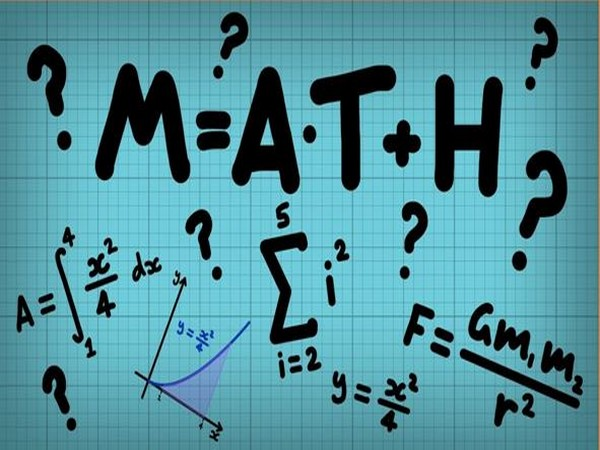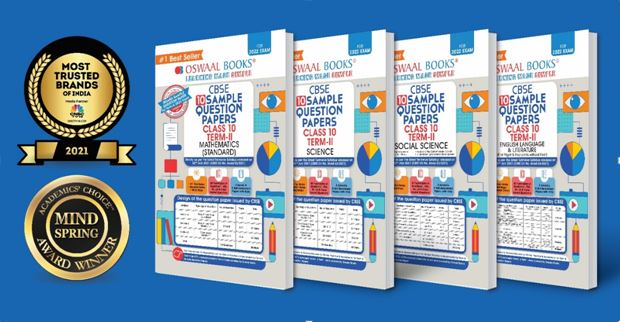हिंदी खबर2 mark questions will be very important for students to crack the Maths exam for class 10.

# CBSE Term 2 2022: Most important questions in Math to go with 2 marks

ANI | Updated: Feb 12, 2022 17:03 IST

New Delhi [India], February 12 (ANI/Oswaal Books): CBSE Class 10 is having an intensely difficult and typical level. The 10 class Maths exam seems to be pretty difficult. If you are a first-time student, it may be tricky to crack the mathematics exam. But if you get the list of some mathematical questions and the statements that will surely come into the exam, it will be very easy for you to crack the exam. Moreover, if you attempt the 2 marks questions, it will be easier for you to crack the exam and score good marks.
We do understand the value of the important questions in mathematics for you. That is why for your ease, we have collected the list of the important question of mathematics to go with the two marks in this article.
Class 10 Term 2 CBSE Board: If you are tired of not getting the important mathematics question, then you may get a solution over here. You can prepare for the latest CBSE Class 10 Maths Term 2 Exam by reviewing these key extremely short answer type questions.
Here you will find chapter-by-chapter relevant two-mark problems to help you prepare for Section A of the Maths paper. There will be six questions (descriptive kind) for 2 points apiece in this section. Practice the crucial questions presented here, which qualified teachers to advise for the term 2 test, to get a perfect score on this section of the paper.
List of important questions:
Note: You may get the two marks questions from the chapter Arithmetic Progression and Circles.
Arithmetic Progressions:
1. How many integers with two digits are divisible by three?
2. The common difference is -4, the seventh term is 4, and the first term is 4.
3. Which of the following terms of AP 8,14,20,26... will be 72 years longer than its 41st term?
4. Write the AP nth term as 1/m, (1+m)/m, (1+2m)/m.
5. Find the phrase in the midst of 6, 13, 20, 216.
Circles:
1. Demonstrate that the circle's diameter comprises line segments connecting the points of contact of two parallel tangents.
2. At C, O is the circle's centre, and BCD is a tangent to it. Demonstrate that BAC+ACD = 900.

3. Demonstrate that the tangents drawn at the endpoints of a circle's diameter are parallel.
4. The centre of two concentric circles is O, with OP=4cm and OB=5cm. At P, AB is an outer circle chord tangent to the inner circle. Calculate the length of AB.
There's only one thing you need for Term-2 Exams to get hands-on results! Read this article to know more!
Tips To Crack 2 Mark Questions of Mathematics in 10:
Read all the equations and the numerical carefully: The first thing that is very important while solving the two marks or any other type of question is reading the question carefully. You should at least try to read the question by two times, and then you can go to attempt the question.
Segregate all your time in the exam: If you attempt all two-mark questions, try your best to segregate and divide the time for all questions. Try to segregate at least half an hour from the whole exam time for two-mark questions.
Keep practising the questions again and again: If you try your best to practice the questions, it will be far better than any other thing you can apply to prepare for your mathematical exam. If you practise the question or the equation, again and again, it will improve your grasping power, and you will also be able to seek all two marks questions in less time. Students can also prepare and Practice with Oswaal CBSE Term 2 Sample Paper Class 10 & 12 For Board Exams 2022. Students will get different ways of learning:
* Self-Assessment Papers for Term 2 Board Exams March-April 2022
* Oswaal CBSE Term 2 Sample Paper Class 10 & 12 For Board Exams 2022 include all latest typologies of Questions as specified in the latest CBSE Sample Papers Released On 14th Jan 2022
* On-Tips Notes & Revision Notes for Quick Revision
* The CBSE Term 2 Sample Paper Class 10 & 12 For Board Exams 2022 include Mind Maps for Better Learning
* The Book provides Free Oswaal 360 E-Assessments based on the latest Typologies of Questions as per CBSE Term 2 Board Exams 2022Here is the recommended link for CBSE Term 2 Sample Paper Class 10 for Board Exams 2022, click here https://bit.ly/3gIHaLu
Here is the recommended link for CBSE Term 2 Sample Paper Class 12 for Board Exams 2022, click here https://bit.ly/3uEO90m
This story is provided by Oswaal Books. ANI will not be responsible in any way for the content of this article. (ANI/Oswaal Books)